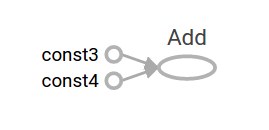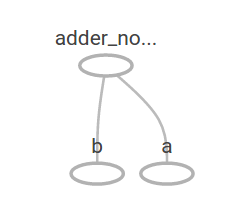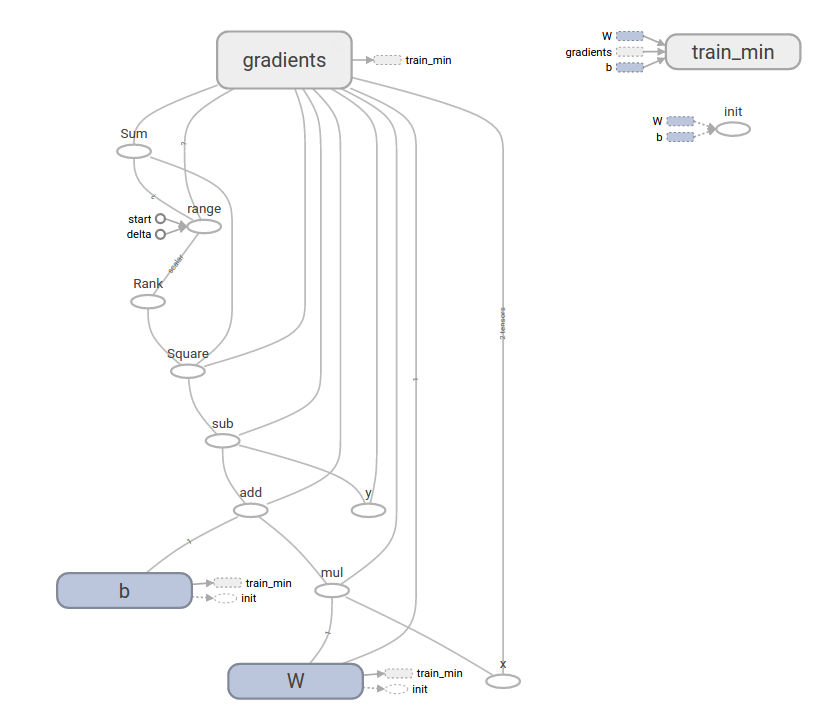https://www.tensorflow.org/

## 一、安装

### 1、安装类型

• 仅支持CPU计算
• 对GPU的支持

#### （2）按运行机制分类

• virtualenv 容器运行
• “native” pip 本地安装
• Docker
• Anaconda

### 2、在Linux（centos）上安装

#### （1）安装Python环境和pip

yum install -y python-pip python-devel # for Python 2.7
yum install -y python34-pip.noarch python34-devel.x86_64 # for Python 2.7

pip -V
pip3 -V

#### （2）安装TensorFlow

pip install tensorflow      # Python 2.7; CPU support (no GPU support)
pip3 install tensorflow     # Python 3.n; CPU support (no GPU support)
pip install tensorflow-gpu  # Python 2.7;  GPU support
pip3 install tensorflow-gpu # Python 3.n; GPU suppor

sudo pip  install --upgrade tfBinaryURL   # Python 2.7
sudo pip3 install --upgrade tfBinaryURL   # Python 3.n

#### （3）验证安装

import tensorflow as tf
hello = tf.constant('Hello, TensorFlow!')
sess = tf.Session()
print(sess.run(hello))

#### （4）卸载

pip uninstall tensorflow  # for Python 2.7
pip3 uninstall tensorflow # for Python 3.n

### 3、在Windows（win10）上安装

#### （1）安装py3.5.2

• 在window上，只支持py3.5.x

#### （2）安装GPU支持CUDA

http://docs.nvidia.com/cuda/cuda-installation-guide-microsoft-windows

http://docs.nvidia.com/cuda/cuda-installation-guide-microsoft-windows/#verify-you-have-cuda-enabled-system

#### （3）安装cuDNN v6.0

https://developer.nvidia.com/cudnn 注册，下载，解压，将dll所在目录加入到Path中（解压目录下\cuda\bin）

#### （4）安装tensorflow

pip3 install --upgrade tensorflow #CPU版
pip3 install --upgrade tensorflow-gpu #GPU版

#### （5）验证安装

python
import tensorflow as tf
hello = tf.constant('Hello, TensorFlow!')
sess = tf.Session()
print(sess.run(hello))

## 二、入门

### 1、TensorFlow入门

TensorFlow 提供两套api

• 低级api是TensorFlow的核心
• 高级api构建于低级api之上，提供更高级的用法，但未稳定

#### （1）Tensors（张量）

TensorFlow中的中心数据单位是Tensors（张量）。张量由一组成形为任意数量的阵列的原始值组成。张量的等级是其维数。以下是张量的一些例子：

3 # 等级0张量;这是一个具有尺寸[]的标量
[1. ,2., 3.] # 等级1张量;这是一个尺寸为的矢量
[[1., 2., 3.], [4., 5., 6.]] # 等级2张量;具有尺寸为[2，3]的矩阵
[[[1., 2., 3.]], [[7., 8., 9.]]] # 3级张量，尺寸为[2,1,3]

### 2、TensorFlow核心教程

#### （1）导入TensorFlow

TensorFlow-Python程序的规范导入声明如下：

import tensorflow as tf

#### （2）计算图

• 构建计算图。
• 运行计算图。

node1 = tf.constant(3.0, dtype=tf.float32) #创建常量张量值为3.0，类型为tf.float32
node2 = tf.constant(4.0) #隐含的类型也为tf.float32
print(node1, node2)

Tensor("Const:0", shape=(), dtype=float32) Tensor("Const_1:0", shape=(), dtype=float32)

sess = tf.Session()
print(sess.run([node1, node2]))

[3.0, 4.0]

ode3 = tf.add(node1, node2)
print("node3: ", node3)
print("sess.run(node3): ",sess.run(node3))

node3:  Tensor("Add:0", shape=(), dtype=float32)
sess.run(node3):  7.0

TensorFlow提供了一个名为TensorBoard的实用程序，可以显示计算图的图片。这是一个屏幕截图，显示TensorBoard如何可视化图形：a = tf.placeholder(tf.float32) #定义一个占位符，元素类型为32位浮点数
b = tf.placeholder(tf.float32)
adder_node = a + b  # + 等价于 tf.add(a, b)

7.5
[ 3.  7.]add_and_triple = adder_node * 3.0 #再附加乘的操作
print(sess.run(add_and_triple, {a: 3, b:4.5})) #执行计算

22.5在机器学习中，我们通常会想要一个可以接受任意输入的模型，比如上面的一个。为了使模型可训练，我们需要能够修改图形以获得具有相同输入的新输出。变量允许我们向图表添加可训练的参数。它们的构造类型和初始值

W = tf.Variable([.3], dtype=tf.float32) #创建一个变量
b = tf.Variable([-.3], dtype=tf.float32)
x = tf.placeholder(tf.float32) #创建一个占位符
linear_model = W * x + b #创建一个线性模型

init = tf.global_variables_initializer()
sess.run(init)

print(sess.run(linear_model, {x:[1,2,3,4]}))

[ 0.          0.30000001  0.60000002  0.90000004]

y = tf.placeholder(tf.float32) #创建占位符
squared_deltas = tf.square(linear_model - y) #求delta^2
loss = tf.reduce_sum(squared_deltas) #求和，计算出最终误差
print(sess.run(loss, {x:[1,2,3,4], y:[0,-1,-2,-3]})) #执行求出损失

23.66

fixW = tf.assign(W, [-1.]) #修改W的值为-1
fixb = tf.assign(b, [1.]) #修改b的值为1
sess.run([fixW, fixb]) #执行更改
print(sess.run(loss, {x:[1,2,3,4], y:[0,-1,-2,-3]})) ##重新计算损失函数

0.0

#### （3）tf.train API

optimizer = tf.train.GradientDescentOptimizer(0.01) #创建梯度下降优化器，学习率为0.01
train = optimizer.minimize(loss) #该优化器为最小化损失函数
sess.run(init) # 将值重置为不正确的默认值
for i in range(1000): #训练1000次
sess.run(train, {x:[1,2,3,4], y:[0,-1,-2,-3]})

print(sess.run([W, b])) #输出训练结果

[array([-0.9999969], dtype=float32), array([ 0.99999082],
dtype=float32)]

import numpy as np
import tensorflow as tf

# Model parameters 模型参数
W = tf.Variable([.3], dtype=tf.float32) #待训练的参数
b = tf.Variable([-.3], dtype=tf.float32)
# Model input and output 模型输入输出
x = tf.placeholder(tf.float32)
linear_model = W * x + b #构建模型
y = tf.placeholder(tf.float32)
# loss 损失函数
loss = tf.reduce_sum(tf.square(linear_model - y)) # sum of the squares
# optimizer 初始化优化器
train = optimizer.minimize(loss)
# training data 加载训练数据
x_train = [1,2,3,4]
y_train = [0,-1,-2,-3]
# training loop 循环训练
init = tf.global_variables_initializer() #加载全局变量初始化器
sess = tf.Session() #获取会话
sess.run(init) # reset values to wrong初始化变量为错误值
for i in range(1000): #循环训练
sess.run(train, {x:x_train, y:y_train})

# evaluate training accuracy 评估培训准确性
curr_W, curr_b, curr_loss = sess.run([W, b, loss], {x:x_train, y:y_train})
print("W: %s b: %s loss: %s"%(curr_W, curr_b, curr_loss))#### （4）tf.contrib.learn

tf.contrib.learn是一个高级TensorFlow库，它简化了机器学习的机制，其中包括：

• 运行训练循环
• 运行评估循环
• 管理数据集
• 管理喂养（managing feeding）

tf.contrib.learn定义了许多常见的模型。

import tensorflow as tf
# NumPy is often used to load, manipulate and preprocess data.
# NumPy通常用于加载，操纵和预处理数据。
import numpy as np

# Declare list of features. We only have one real-valued feature. There are many
# other types of columns that are more complicated and useful.
# 声明特征列表。我们只有一个实数的特征。
# 当然还有其他的更加复杂和有用的列类型
features = [tf.contrib.layers.real_valued_column("x", dimension=1)]

# An estimator is the front end to invoke training (fitting) and evaluation
# (inference). There are many predefined types like linear regression,
# logistic regression, linear classification, logistic classification, and
# many neural network classifiers and regressors. The following code
# provides an estimator that does linear regression.
# 估计器是一个实现训练和评估的前端。有许多预定义类型，
# 如线性回归， 逻辑回归，线性分类，物流分类和很多神经网络分类器和回归器。
# 以下代码提供一个进行线性回归的估计
estimator = tf.contrib.learn.LinearRegressor(feature_columns=features)

# TensorFlow provides many helper methods to read and set up data sets.
# Here we use two data sets: one for training and one for evaluation
# We have to tell the function how many batches
# of data (num_epochs) we want and how big each batch should be.
# TensorFlow提供了许多帮助方法来读取和设置数据集。
# 这里我们使用两个数据集：一个用于训练，一个用于评估
# 我么还有告诉函数有多少批次数据(num_epochs)，每一批次数据有多大
x_train = np.array([1., 2., 3., 4.]) #训练集数据
y_train = np.array([0., -1., -2., -3.])
x_eval = np.array([2., 5., 8., 1.]) #验证集数据
y_eval = np.array([-1.01, -4.1, -7, 0.])
input_fn = tf.contrib.learn.io.numpy_input_fn({"x":x_train}, y_train,
batch_size=4,
num_epochs=1000)
eval_input_fn = tf.contrib.learn.io.numpy_input_fn(
{"x":x_eval}, y_eval, batch_size=4, num_epochs=1000)

# We can invoke 1000 training steps by invoking the  method and passing the
# training data set.
# 我们可以通过调用方法和传递训练数据集来实现1000此训练。
estimator.fit(input_fn=input_fn, steps=1000)

# Here we evaluate how well our model did.
# 在这里我们评估我们的模型做得如何。
train_loss = estimator.evaluate(input_fn=input_fn)
eval_loss = estimator.evaluate(input_fn=eval_input_fn)
print("train loss: %r"% train_loss)
print("eval loss: %r"% eval_loss)

train loss: {'loss': 2.0180224e-08, 'global_step': 1000}
eval loss: {'loss': 0.0025395651, 'global_step': 1000}

tf.contrib.learn不会将您锁定到其预定义的模型中。假设我们想创建一个没有内置到TensorFlow中的自定义模型。我们仍然可以保留tf.contrib.learn的数据集，饲养，培训等的高级抽象。为了说明，我们将使用我们对较低级别TensorFlow API的了解，展示如何使用LinearRegressor实现我们自己的等效模型。

import numpy as np
import tensorflow as tf
# Declare list of features, we only have one real-valued feature

def model(features, labels, mode):
# Build a linear model and predict values
# 构建一个线性回归模型和预测值
W = tf.get_variable("W", , dtype=tf.float64)
b = tf.get_variable("b", , dtype=tf.float64)
y = W*features['x'] + b
# Loss sub-graph
# 损失函数子图
loss = tf.reduce_sum(tf.square(y - labels))
# Training sub-graph
# 训练子图
global_step = tf.train.get_global_step()
train = tf.group(optimizer.minimize(loss),
# ModelFnOps connects subgraphs we built to the
# appropriate functionality.
# ModelFnOps将我们构建的子图连接到适当的功能。
return tf.contrib.learn.ModelFnOps(
mode=mode, predictions=y,
loss=loss,
train_op=train)

estimator = tf.contrib.learn.Estimator(model_fn=model)
# define our data sets
# 定义数据集
x_train = np.array([1., 2., 3., 4.])
y_train = np.array([0., -1., -2., -3.])
x_eval = np.array([2., 5., 8., 1.])
y_eval = np.array([-1.01, -4.1, -7, 0.])
input_fn = tf.contrib.learn.io.numpy_input_fn({"x": x_train}, y_train, 4, num_epochs=1000)
eval_input_fn = tf.contrib.learn.io.numpy_input_fn({"x": x_eval}, y_eval, 4, num_epochs=1000)

# train
# 训练
estimator.fit(input_fn=input_fn, steps=1000)
# Here we evaluate how well our model did.
# 这儿我们将评估我们的模型表现如何
train_loss = estimator.evaluate(input_fn=input_fn)
eval_loss = estimator.evaluate(input_fn=eval_input_fn)
print("train loss: %r"% train_loss)
print("eval loss: %r"% eval_loss)

train loss: {'global_step': 1000, 'loss': 6.4150553e-11}
eval loss: {'global_step': 1000, 'loss': 0.010101151}

### 3、MNIST机器学习入门

#### （1）MNIST数据集

MNIST是一个入门级的计算机视觉数据集，它包含各种手写数字图片（大小为28*28），它也包含每一张图片对应的标签，告诉我们这个是数字几。

TensorFlow提供了自动下载读取该数据集的函数：

from tensorflow.examples.tutorials.mnist import input_data
#源码参见：https://tensorflow.googlesource.com/tensorflow/+/master/tensorflow/examples/tutorials/mnist/input_data.py

• mnist.train.images为尺寸为[60000, 784]的张量，60000表示有60000张图片，748（28*28）表示一张图片，在此张量里的每一个元素，都表示某张图片里的某个像素的强度值，值介于0和1之间。
• mnist.train.labels是一个 [60000, 10] 的数字矩阵，表示对应图片的标签
• 测试集类似

#### （2）Softmax回归介绍

softmax回归（softmax regression）分两步：第一步

#### （3）实现回归模型

TensorFlow也把复杂的计算放在python之外完成，但是为了避免前面说的那些开销，它做了进一步完善。Tensorflow不单独地运行单一的复杂计算，而是让我们可以先用图描述一系列可交互的计算操作，然后全部一起在Python之外运行。（这样类似的运行方式，可以在不少的机器学习库中看到。）

import tensorflow as tf

x = tf.placeholder("float", [None, 784])

x不是一个特定的值，而是一个占位符placeholder，我们在TensorFlow运行计算时输入这个值。我们希望能够输入任意数量的MNIST图像，每一张图展平成784维的向量。我们用2维的浮点数张量来表示这些图，这个张量的形状是[None，784 ]。（这里的None表示此张量的第一个维度可以是任何长度的。）

W = tf.Variable(tf.zeros([784,10]))
b = tf.Variable(tf.zeros())

y = tf.nn.softmax(tf.matmul(x,W) + b)

#### （4）训练模型

y_ = tf.placeholder("float", [None,10])

cross_entropy = tf.reduce_mean(-tf.reduce_sum(y_ * tf.log(y), reduction_indices=))

train_step = tf.train.GradientDescentOptimizer(0.01).minimize(cross_entropy)

TensorFlow在这里实际上所做的是，它会在后台给描述你的计算的那张图里面增加一系列新的计算操作单元用于实现反向传播算法和梯度下降算法。然后，它返回给你的只是一个单一的操作，当运行这个操作时，它用梯度下降算法训练你的模型，微调你的变量，不断减少成本。

init = tf.initialize_all_variables()

sess = tf.Session()
sess.run(init)

for i in range(1000):
batch_xs, batch_ys = mnist.train.next_batch(100)
sess.run(train_step, feed_dict={x: batch_xs, y_: batch_ys})

#### （5）评估我们的模型

correct_prediction = tf.equal(tf.argmax(y,1), tf.argmax(y_,1))

accuracy = tf.reduce_mean(tf.cast(correct_prediction, "float"))

print sess.run(accuracy, feed_dict={x: mnist.test.images, y_: mnist.test.labels})

### 4、MNIST机器学习深入

### 步骤1：加载数据 ###
from tensorflow.examples.tutorials.mnist import input_data

# 查看数据
# mnist.train.images为训练数据
print(mnist.train.images.ndim) #获取数据维度
print(mnist.train.images.shape) #获取数组各个维度的大小
print(mnist.train.labels.shape)

# 引入tf库
import tensorflow as tf
sess = tf.InteractiveSession() #创建交互式会话

### 步骤2：创建输入训练样本数据和标签的占位符 ###

# 创建训练数据所用的占位符，维度为2，第一维为任意在运行时指定，
# 取决于批次batch
x = tf.placeholder("float", shape=[None, 784]) #输入占位符
y_ = tf.placeholder("float", shape=[None, 10]) #数据标签（输出）占位符

### 步骤3：创建模型参数 ###
# 创建训练特征变量（权重W和偏置b）

# 创建权重的函数
def weight_variable(shape):
initial = tf.truncated_normal(shape, stddev=0.1)
return tf.Variable(initial)

# 创建偏置的的函数
def bias_variable(shape):
initial = tf.constant(0.1, shape=shape)
return tf.Variable(initial)

#第一层卷积的参数
W_conv1 = weight_variable([5, 5, 1, 32]) #5*5滤波器，输入通道为1，输出通道为32
b_conv1 = bias_variable()
#第二层卷积的参数
W_conv2 = weight_variable([5, 5, 32, 64])
b_conv2 = bias_variable()
#密集连接层的参数
W_fc1 = weight_variable([7 * 7 * 64, 1024])
b_fc1 = bias_variable()
#输出层的参数
W_fc2 = weight_variable([1024, 10])
b_fc2 = bias_variable()

### 步骤4：构建假设函数模型y ###
# 卷积和池化函数
def conv2d(x, W):
return tf.nn.conv2d(x, W, strides=[1, 1, 1, 1], padding='SAME')

def max_pool_2x2(x):
return tf.nn.max_pool(x, ksize=[1, 2, 2, 1],

# 构建y的模型
#对输入x重塑成1通道的张量
x_image = tf.reshape(x, [-1,28,28,1])
# 第一层卷积和池化降维
# 公式为：ReLU激活(卷积(x,w) + b)
#输入：1*28*28 输出为32*14*14
h_conv1 = tf.nn.relu(conv2d(x_image, W_conv1) + b_conv1)
h_pool1 = max_pool_2x2(h_conv1) #2*2降维，
# 第二层卷积和池化降维
#输入：32*14*14 输出为：64*7*7
h_conv2 = tf.nn.relu(conv2d(h_pool1, W_conv2) + b_conv2)
h_pool2 = max_pool_2x2(h_conv2)
#密集连接层
h_pool2_flat = tf.reshape(h_pool2, [-1, 7*7*64]) #展开64*7*7的张量
h_fc1 = tf.nn.relu(tf.matmul(h_pool2_flat, W_fc1) + b_fc1)
#Dropout层，防止过拟合
keep_prob = tf.placeholder("float")
h_fc1_drop = tf.nn.dropout(h_fc1, keep_prob)
#输出层
y_conv=tf.nn.softmax(tf.matmul(h_fc1_drop, W_fc2) + b_fc2)

### 步骤5：构造代价函数 ###
cross_entropy = tf.reduce_mean(
tf.nn.softmax_cross_entropy_with_logits(labels=y_, logits=y_conv))

### 步骤6：训练模型 ###

correct_prediction = tf.equal(tf.argmax(y_conv, 1), tf.argmax(y_, 1))
accuracy = tf.reduce_mean(tf.cast(correct_prediction, tf.float32))

#初始化、训练
with tf.Session() as sess:
sess.run(tf.global_variables_initializer())
for i in range(20000):
batch = mnist.train.next_batch(50)
if i % 100 == 0:
train_accuracy = accuracy.eval(feed_dict={
x: batch, y_: batch, keep_prob: 1.0})
print('step %d, training accuracy %g' % (i, train_accuracy))
train_step.run(feed_dict={x: batch, y_: batch, keep_prob: 0.5})
### 步骤7：评估模型 ###
print(sess.run(accuracy, feed_dict={x: mnist.test.images, y_: mnist.test.labels}))

### 5、tf.contrib.learn快速开始

#### （1）描述

• 以下使用tf的高级api完成深度神经网络（DNN）的构建、训练、评价
• 问题：为对三种花的分类
• 数据：格式为cvs，每行为花的参数（萼片长度，萼片宽度，花瓣长度，花瓣宽度，和花的品种）

#### （2）python3代码

import os
import urllib.request

import numpy as np
import tensorflow as tf

# 数据集
IRIS_TRAINING = "iris_training.csv"

IRIS_TEST = "iris_test.csv"

# 主函数
def main():
### 下载训练和测试集 ###
# 如果训练集和测试集没有不存在，下载它们。
if not os.path.exists(IRIS_TRAINING):
#    print(type(raw))
#    print(len(raw))
#    print(raw)
with open(IRIS_TRAINING, "wb") as f:
f.write(raw)

if not os.path.exists(IRIS_TEST):
with open(IRIS_TEST, "wb") as f:
f.write(raw)
### 加载训练和测试集 ###
# 载入数据集。
filename=IRIS_TRAINING, #文件名
target_dtype=np.int, #标签的数据类型
features_dtype=np.float32) #输入特征的数据类型

# print(training_set)

filename=IRIS_TEST,
target_dtype=np.int,
features_dtype=np.float32)

### 构建深度神经网络分类器 ###
# 指定训练集特征的数目和数据类型
feature_columns = [tf.contrib.layers.real_valued_column("", dimension=4)]

# 构建3层DNN（深度神经网络，全连接），分别为10,20,10个节点。
classifier = tf.contrib.learn.DNNClassifier(feature_columns=feature_columns,
hidden_units=[10, 20, 10],
n_classes=3,
model_dir="/tmp/iris_model")
### 描述训练输入管道 ###
def get_train_inputs():
x = tf.constant(training_set.data)
y = tf.constant(training_set.target)

return x, y

### 将模型连接到数据 ###
classifier.fit(input_fn=get_train_inputs, steps=2000)

# 定义测试输入数据x和标签y
def get_test_inputs():
x = tf.constant(test_set.data)
y = tf.constant(test_set.target)

return x, y

### 评估模型精度 ###
# 评估准确性
accuracy_score = classifier.evaluate(input_fn=get_test_inputs,
steps=1)["accuracy"]

print("\nTest Accuracy: {0:f}\n".format(accuracy_score))

### 应用训练好的模型 ###
# 分类两个新鲜花样本。
def new_samples():
return np.array(
[[6.4, 3.2, 4.5, 1.5],
[5.8, 3.1, 5.0, 1.7]], dtype=np.float32)

predictions = list(classifier.predict(input_fn=new_samples))

print(
"New Samples, Class Predictions:    {}\n"
.format(predictions))

if __name__ == "__main__":
main()

#### （3）程序流程

• filename，文件位置
• target_dtype，数据集的目标值（标签）的numpy数据类型
• features_dtype, 它使用数据集的特征值（特征）的numpy数据类型。

tf.contrib.learn 数据集表示为namedtuple的形式，您可以通过数据和目标字段访问特征数据和目标值。这里，training_set.datatraining_set.target分别包含训练集的特征数据和目标值，test_set.data和test_set.target包含测试集的要素数据和目标值。

tf.contrib.learn提供了各种预定义的模型，称为估算器（ Estimators），您可以使用“开箱即用”来对数据运行培训和评估操作。在这里，您将配置深层神经网络分类器模型以适应Iris数据。使用tf.contrib.learn，您可以使用几行代码实例化tf.contrib.learn.DNNClassifier

• 首先定义特征列的数目和数据类型，tf.contrib.layers.real_valued_column是用于构建要素列的适当函数。
• 然后tf.contrib.learn.DNNClassifier使用以下参数创建
• feature_columns=feature_columns
• hidden_units=[10, 20, 10]：三个隐藏层，分别含有10,20和10个神经元。
• n_classes = 3：三个目标分类器，代表三分类问题。
• model_dir=/tmp/iris_model：TensorFlow将在模型训练期间保存检查点数据的目录。有关使用TensorFlow进行日志和监视的更多信息，请看：Logging and Monitoring Basics with tf.contrib.learn

tf.contrib.learnAPI使用输入函数来为模型提供训练数据。在这个例子中，数据足够小，可以存储在TensorFlow常量中。以下代码生成最简单的输入管道：

# Define the training inputs
def get_train_inputs():
x = tf.constant(training_set.data)
y = tf.constant(training_set.target)

return x, y

classifier.fit(x=training_set.data, y=training_set.target, steps=1000)
classifier.fit(x=training_set.data, y=training_set.target, steps=1000)